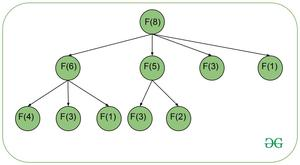# Count of distinct Primonacci Numbers in a given range [L, R]

Given a range [L, R], the task is to count the Primonacii Number present in that given range. Primonacii numbers are the part of Primonacii Series, defined below:

Primonacii Series is defined as

F(1) = F(2) = 1

F(N) = F(N – 2) + F(N – 3) + F(N – 5) + …… + F(N – K), where K is the nearest prime number smaller than N.

The first few terms of the series are 1, 1, 1, 2, 2, 4, 5, 8, 12 …

Examples:

Input: L = 2, R = 7

Output: 3

Explanation:

All primonacii numbers in range [2, 7] are {2, 4, 5}.

Input: L = 6, R = 7

Output: 0

Explanation:

There are no primonacii numbers in given range.

Naive Approach:

The idea is to firstly use Sieve Of Eratosthenes to find all prime numbers up to a maximum number. Now, use Recursion to generate the Primonacii Series up to R by solving the complete recurrence relation, which will take exponential time. Finally, traverse the series and count the number of elements which falls in range [L, R].

Time Complexity: O(2N)

Auxiliary Space: O(N)

Efficient Approach:

While generating Primonacii Series up to a given number N = 8, the partial recursion tree would look like:Here, it can be observed that various sub-problems like F(3) and F(1) are solved multiple times. These overlapping sub-problems can be overcome using Dynamic Programming, which ensures that such sub-problems will be solved only once. In this way, the primonacii series can be generated in linear time. Once the series is obtained, traverse over the series and count the number of elements in range [L, R]. Below are the steps to solve this problem:

• Build a Sieve of maximum size M and find all primes up to M. Refer this article to check how to build sieve.
• Create an array dp[ ], where dp[i] will store the ith Primonacii Number. Set dp = dp = 1.
• Iterate over all primes P, which are less than N.
• For each P, add the (N-K)th Primonacii number stored at dp[N-K] to dp[i].
• Finally, traverse the array dp[ ] and count the elements which falls in range [L, R].

Below is the implementation of the above approach:

## Java

 `// Java Program to implement` `// the above appraoch` `import` `java.util.*;` `class` `GFG {`   `    ``// Assumed maximum number` `    ``static` `int` `M = ``100005``;` `  `  `    ``// List of primes` `    ``static` `ArrayList primes;`   `    ``// Function to find` `    ``// all primes` `    ``static` `void` `sieve()` `    ``{` `        ``primes = ``new` `ArrayList();`   `        ``// To mark the prime ones` `        ``boolean` `mark[] = ``new` `boolean``[M];`   `        ``// Initially all marked as prime` `        ``for` `(``int` `i = ``2``; i < M; i++)` `            ``mark[i] = ``true``;`   `        ``for` `(``int` `i = ``2``; i * i < M; i++) {`   `            ``// If i is prime` `            ``if` `(mark[i]) {`   `                ``// Mark all multiples` `                ``// of i as non-prime` `                ``for` `(``int` `j = i * i; j < M; ` `                                   ``j += i)`   `                    ``mark[j] = ``false``;` `            ``}` `        ``}`   `        ``// Adding all primes to a list` `        ``for` `(``int` `i = ``2``; i < M; i++)` `            ``if` `(mark[i])` `                ``primes.add(i);` `    ``}`   `    ``// Function returns the count of` `    ``// primonacii numbers in given range` `    ``static` `int` `countPrimonacii(``int` `l, ``int` `r)` `    ``{`   `        ``// Generating the series` `        ``// here dp[i] denotes ith` `        ``// primonacci number` `        ``int` `dp[] = ``new` `int``[r + ``1``];` `        ``dp[``1``] = dp[``2``] = ``1``;`   `        ``for` `(``int` `i = ``3``; i <= r; i++) {`   `            ``for` `(``int` `j = ``0``; j < primes.size(); ` `                                        ``j++) {`   `                ``int` `p = primes.get(j);`   `                ``if` `(p >= i)` `                    ``break``;`   `                ``dp[i] += dp[i - p];` `            ``}` `        ``}`   `        ``int` `count = ``0``;`   `        ``// First three terms are 1` `        ``// so to count it only once` `        ``if` `(l <= ``1` `&& r >= ``1``)` `            ``count++;`   `        ``// Fourth and fifth term are 2` `        ``// so to count it only once` `        ``if` `(l <= ``2` `&& r >= ``2``)` `            ``count++;`   `        ``// Now onwards, all terms are unique` `        ``// check if they fall in the range` `        ``for` `(``int` `i = ``6``; i <= r; i++) {` `            ``if` `(dp[i] >= l && dp[i] <= r)` `                ``count++;` `        ``}`   `        ``return` `count;` `    ``}`   `    ``// Driver Code` `    ``public` `static` `void` `main(String[] args)` `    ``{` `        ``// Building sieve` `        ``sieve();`   `        ``int` `l = ``2``, r = ``7``;`   `        ``System.out.println(countPrimonacii(l, r));` `    ``}` `}`

Output

```3
```

Time Complexity: O(M * log(log M))) where M is the maximum size of the sieve.

Auxiliary Space: O(M)

Attention reader! Don’t stop learning now. Get hold of all the important DSA concepts with the DSA Self Paced Course at a student-friendly price and become industry ready.

My Personal Notes arrow_drop_upCheck out this Author's contributed articles.

If you like GeeksforGeeks and would like to contribute, you can also write an article using contribute.geeksforgeeks.org or mail your article to contribute@geeksforgeeks.org. See your article appearing on the GeeksforGeeks main page and help other Geeks.

Please Improve this article if you find anything incorrect by clicking on the "Improve Article" button below.